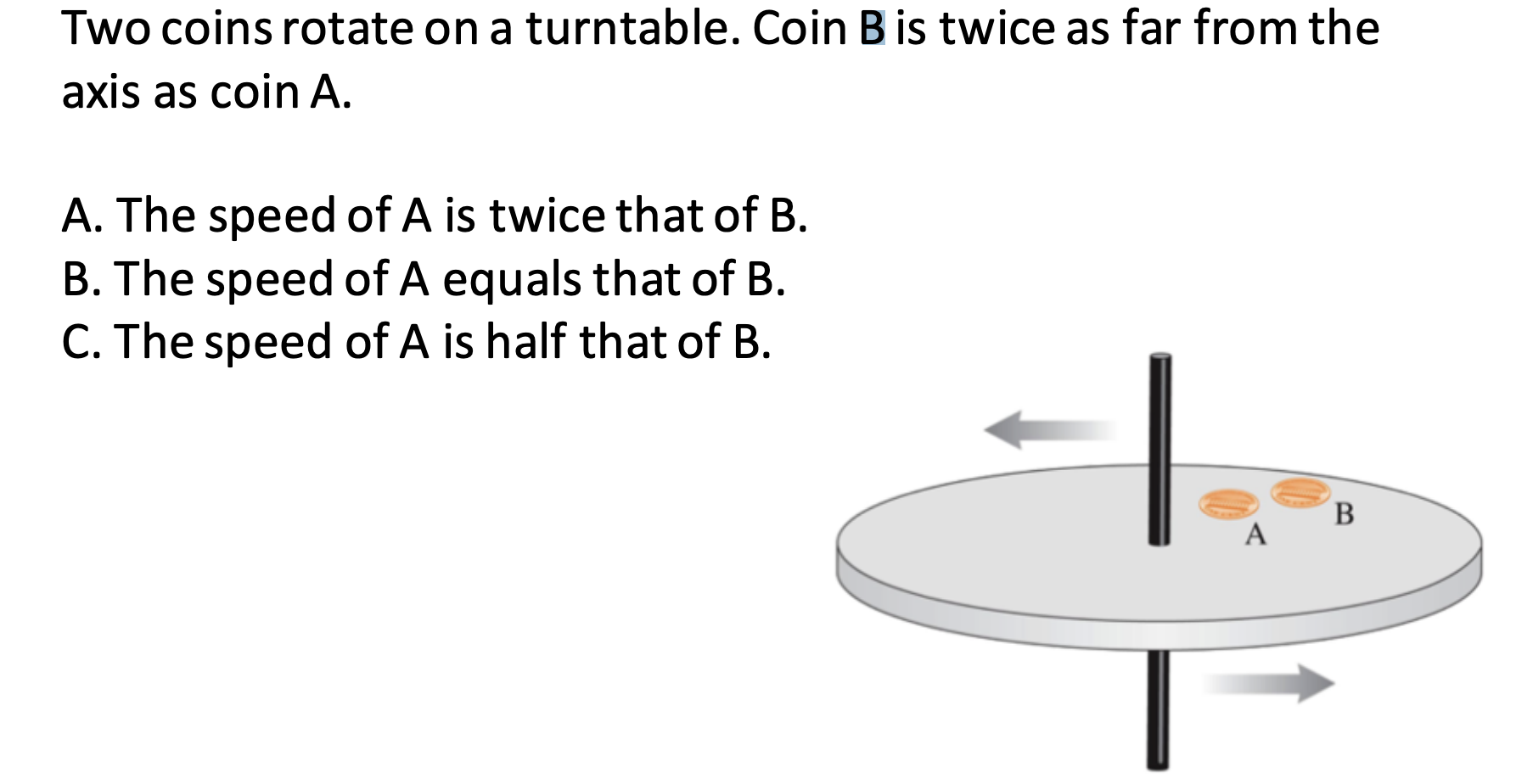# Two coins rotate on a turntable. Coin B is twice as far from the axis as coin A A. The speed of A is twice that of B B. The speed of A equals that of B C. The speed of A is half that of B В

Question

Is the speed of A 2x as fast as B?help_outlineImage TranscriptioncloseTwo coins rotate on a turntable. Coin B is twice as far from the axis as coin A A. The speed of A is twice that of B B. The speed of A equals that of B C. The speed of A is half that of B В fullscreen

### Want to see this answer and more?

Experts are waiting 24/7 to provide step-by-step solutions in as fast as 30 minutes!*